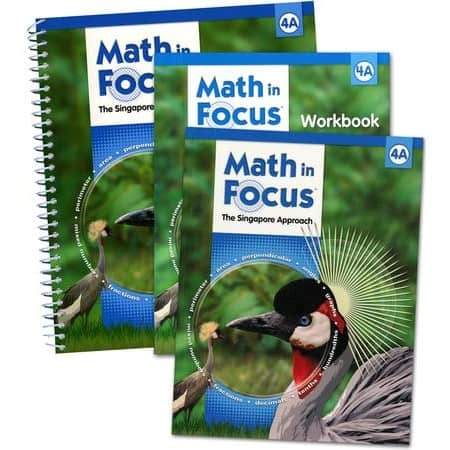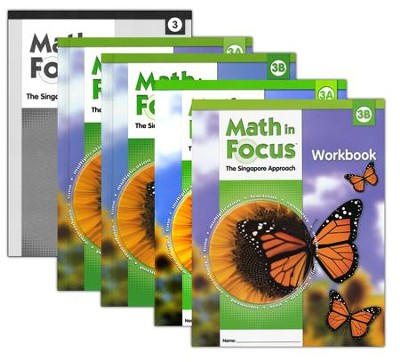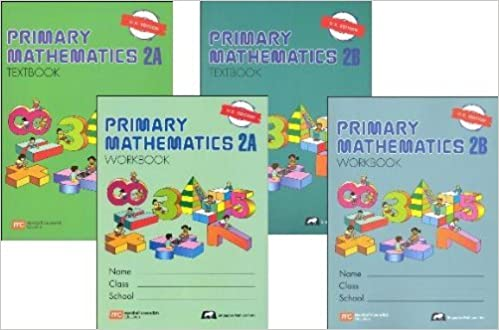Singapore Math

# Singapore Math: Math in Focus Vs Primary Mathematics Vs Dimensions Math

Math in Focus, Primary Mathematics and Dimensions Math are three Singapore Mathematics curricula used in schools and homeschooling families in the United States. In this post, we review these three different curricula for Grades 1 to 5.

# Math in Focus Vs Primary Mathematics Vs Dimensions Math

## Math in Focus

Math in Focus (MIF) is the U.S. version of Singapore’s mathematics program My Pals are Here! Math in Focus is distributed in the U.S. through

The Math in Focus curriculum covers Grades K to 8. Math in Focus offers comprehensive material to support schools and homeschool students. Math in Focus offers material and resources to support differentiated learning. Math in Focus is also available in Spanish.

Math in Focus has come up with a revised version for 2020. In the latest revision, Math in Focus © 2020, is said to be the program’s most extensive revision since its  2009 release.

The latest version can be purchased from the publisher’s website. Printed material is not yet widely available from other online retailers for the 2020 edition.

In summary, the resources available for Math in Focus are:

#### Math in Focus Homeschool

In addition to printed material, the Math in Focus curriculum provides online resources for homeschoolers.

Students can sign up for a preview of the latest 2020 edition on the publisher’s website.

##The cost of adopting Math in Focus as your homeschool curriculum would vary according to the materials you would get.  Math in Focus provides the most resources available for homeschoolers as compared to the other two curricula. The teacher’s guide compared to the other two curricula is the most expensive. The prices for the textbooks and workbooks would vary depending on the edition. Parents can also find good bargains for second-hand material on eBay or Amazon. Buying in bundles can also result in savings.

The cost of 2 teacher’s guides (A & B), 2 textbooks (A & B), and 2 workbooks (A & B) could cost approximately \$250 to \$300 for editions prior to 2020.## Primary Mathematics

There are three editions to Primary Mathematics to serve the diverse needs of the students and schools in the United States. Primary Mathematics was adapted to the U.S. market by Singapore Math, Inc.

#### Primary Mathematics U.S. Edition (Grades 1 – 6)

Primary Mathematics U.S. edition was modeled after the original program used in Singapore years ago, but with U.S. measurements and currency.

In summary, the resources available for Primary Mathematics U.S. Edition Grades 1 to 6 are:

#### Primary Mathematics Standards Edition (Grades 1 – 6)

The Primary Mathematics Standards edition was written to meet the 2006 Mathematics Contents Standards for California Public School issued prior to the Common Core State Standards.

In summary, the resources available for Primary Mathematics U.S. Edition Grades 1 to 6 are:

#### Primary Mathematics Common Core Edition (Grades 1 – 5)

The Primary Mathematics Common Core edition was written to align with Common Core State Standards.

In summary, the resources available for Primary Mathematics U.S. Edition Grades 1 to 5 are:

#### Primary Mathematics Homeschool

Primary Mathematics is pretty popular with homeschoolers since it was the first Singapore Math curriculum available for the U.S. market. A home instructor’s guide is available for Primary Mathematics to help parents implement the curriculum.

The cost of implementation is pretty reasonable. The cost of 2 textbooks (A & B), 2 workbooks (A & B), and 2 instructor’s/ teacher’s guide (A & B) would cost approximately \$100 to \$200. Costs would depend on the edition chosen.## Dimensions Math

Dimensions Math is published by Singapore Math Inc. and was designed to cater to the needs of U.S. teachers and students and said to be a sequel to Primary Mathematics. The Dimensions Math program explains concepts in a new format as compared to Primary Mathematics. The Dimensions Math curriculum covers grades PK to 8.

In summary, the resources available for Dimensions Math are:

#### Dimensions Math Homeschool

Dimensions Math is the newest publication compared to Math in Focus and Primary Mathematics. The cost of implementation is very reasonable. The cost of 2 textbooks (A & B), 2 workbooks (A & B), and 2 teacher’s guides (A & B) cost approximately \$100.

## Chapters and Sequence Comparison

Below are the chapters covered and the sequence of chapters.  The different curricula arrange and title their topics differently. For more detailed information on the details of what is covered in each chapter, refer to the individual curriculum material.

## Singapore Mathematics Curriculum Comparison Grade 1

wdt_IDMath in FocusMath in Focus 2020Primary Mathematics US EditionPrimary Mathematics Standards EditionPrimary Mathematics Common Core EditionDimensions Math
1Chapter 1: Numbers to 10 (1A)Chapter 1: Numbers to 10Chapter 1: Numbers 0 to 10 (1A)Chapter 1: Numbers 0 to 10 (1A)Chapter 1: Numbers 0 to 10 (1A)Chapter 1: Numbers to 10 (1A)
2Chapter 2: Number Bonds.Chapter 2: Addition and Subtraction Within 10Chapter 2: Number BondsChapter 2: Number BondsChapter 2: Number BondsChapter 2: Number Bonds
4Chapter 4: Subtraction Facts to 10Chapter 4: Numbers to 20Chapter 4: SubtractionChapter 4: SubtractionChapter 4: Subtraction Within 10Chapter 4: Subtraction
5Chapter 5: Shapes and PatternsChapter 5: Addition and Subtraction Within 20Chapter 5: Ordinal NumbersChapter 5: PositionChapter 5: Ordinal NumbersChapter 5: Numbers to 20
6Chapter 6: Ordinal Numbers and PositionChapter 6: Numbers to 40Chapter 6: Numbers to 20Chapter 6: Numbers to 20Chapter 6: Numbers to 20Chapter 6: Addition to 20
7Chapter 7: Numbers to 20Chapter 7: Calendar and TimeChapter 7: ShapesChapter 7: ShapesChapter 7: ShapesChapter 7: Subtraction Within 20
8Chapter 8: Addition and Subtraction Facts to 20Chapter 8: Addition and Subtraction Within 40Chapter 8: LengthChapter 8: LengthChapter 8: LengthChapter 8: Shapes
9Chapter 9: LengthChapter 9: Length and WeightChapter 9: WeightChapter 9: WeightChapter 9: Comparing NumbersChapter 9: Ordinal Numbers
10Chapter 10: Weight (1B)Chapter 10: Numbers to 120Chapter 10: Comparing Numbers (1B)Chapter 10: CapacityChapter 10: GraphsChapter 10: Length (1B)

## Singapore Mathematics Curriculum Comparison Grade 2

wdt_IDMath in FocusMath in Focus 2020Primary Mathematics US EditionPrimary Mathematics Standards EditionPrimary Mathematics Common Core EditionDimensions Math
1Chapter 1: Numbers to 1,000 (2A)Chapter 1: Numbers to 1,000Chapter 1: Numbers to 1,000 (2A)Chapter 1: Numbers to 1,000 (2A)Chapter 1: Numbers to 1,000 (2A)Chapter 1: Numbers to 1,000 (2A)
3Chapter 3: Subtraction up to 1,000Chapter 3: Subtraction Within 1,000Chapter 3: LengthChapter 3: LengthChapter 3: LengthChapter 3: Addition and Subtraction Part 2
4Chapter 4: Using Bar Models: Addition and SubtractionChapter 4: Using Bar Models: Addition and SubtractionChapter 4: WeightChapter 4: WeightChapter 4: Multiplication and DivisionChapter 4: Length
5Chapter 5: Multiplication and DivisionChapter 5: LengthChapter 5: Multiplication and DivisionChapter 5: Multiplication and DivisionChapter 5: Multiplication Tables of 2 and 3Chapter 5: Weight
6Chapter 6: Multiplication Tables of 2, 5, and 10Chapter 6: MassChapter 6: Multiplication Tables of 2 and 3Chapter 6: Multiplication Tables of 2 and 3Chapter 6: Addition and Subtraction (2B)Chapter 6: Multiplication and Division
7Chapter 7: Metric Measurement of LengthChapter 7: Graphs and Line PlotsChapter 7: Addition and Subtraction (2B)Chapter 7: Addition and Subtraction (2B)Chapter 7: Multiplication and Division (4, 5 and 10)Chapter 7: Multiplication and Division of 2, 5 and 10
8Chapter 8: MassChapter 8: Multiplication and DivisionChapter 8: Multiplication and Division (4, 5 and 10)Chapter 8: Multiplication and Division (4, 5, and 10)Chapter 8: MoneyChapter 8: Mental Calculation
9Chapter 9: VolumeChapter 9: Multiplication Tables (2, 5, 10, 3 and 4)Chapter 9: MoneyChapter 9: MoneyChapter 9: FractionsChapter 9: Multiplication and Division of 3 and 4
10Chapter 10: Mental Math and EstimationChapter 10: Time and MoneyChapter 10: FractionsChapter 10: FractionsChapter 10: TimeChapter 10: Money

## Singapore Mathematics Curriculum Comparison Grade 3

wdt_IDMath in FocusMath in Focus 2020Primary Mathematics US EditionPrimary Mathematics Standards EditionPrimary Mathematics Common Core EditionDimensions Math
1Chapter 1: Numbers to 10,000 (3A)Chapter 1: Numbers to 10,000Chapter 1: Numbers to 10,000 (3A)Chapter 1: Numbers to 10,000 (3A)Chapter 1: Numbers to 10,000Chapter 1: Numbers to 10,000
2Chapter 2: Mental Math and EstimationChapter 2: Addition Within 10,000Chapter 2: Addition and SubtractionChapter 2: Addition and SubtractionChapter 2: Addition and SubtractionChapter 2: Addition and Subtraction Part 1
3Chapter 3: Addition to to 10,000Chapter 3: Subtraction Within 10,000Chapter 3: Multiplication and DivisionChapter 3: Multiplication and DivisionChapter 3: Multiplication and DivisionChapter 3: Addition and Subtraction Part 2
4Chapter 4: Subtraction up to 10,000Chapter 4: Multiplication Tables (6, 7, 8, 9, 11 and 12)Chapter 4: Multiplication Tables of 6, 7, 8 and 9Chapter 4: Multiplication Tables of 6, 7, 8 and 9Chapter 4: Multiplication Tables of 6, 7, 8 and 9Chapter 4: Multiplication and Division
5Chapter 5: Using Bar Models: Addition and SubtractionChapter 5: MultiplicationChapter 5: MoneyChapter 5: Data Analysis (Presenting Data, Probability)Chapter 5: LengthChapter 5: Multiplication
6Chapter 6: Multiplication Tables of 6, 7, 8 and 9Chapter 6: Using Bar Models: The Four OperationsChapter 6: Mental Calculation (3B)Chapter 6: Length (3B)Chapter 6: Mass and Weight (3B)Chapter 6: Division
7Chapter 7: MultiplicationChapter 7: FractionsChapter 7: LengthChapter 7: WeightChapter 7: CapacityChapter 7: Graphs and Tables
8Chapter 8: DivisionChapter 8: MeasurementChapter 8: WeightChapter 8: CapacityChapter 8: MoneyChapter 8: Multiplying and Dividing with 6, 7, 8, and 9
9Chapter 9: Using Bar Models: Multiplication and DivisionChapter 9: Area and PerimeterChapter 9: CapacityChapter 9: MoneyChapter 9: FractionsChapter 9: Fractions Part 1
10Chapter 10: Money (3B)Chapter 10: TimeChapter 10: GraphsChapter 10: FractionsChapter 10: TimeChapter 10: Fractions Part 2

## Singapore Mathematics Curriculum Comparison Grade 4

wdt_IDMath in FocusMath in Focus 2020Primary Mathematics US EditionPrimary Mathematics Standards EditionPrimary Mathematics Common Core EditionDimensions Math
1Chapter 1: Working With Whole Numbers to 100,000 (4A)Chapter 1: Working With Whole Numbers (to 1,000,000)Chapter 1: Whole Numbers to 100,000 (4A)Chapter 1: Whole Numbers (4A)Chapter 1: Numbers to 1,000,000 (4A)Chapter 1: Numbers to One Million (4A)
2Chapter 2: Estimation and Number TheoryChapter 2: Multiplication and DivisionChapter 2: Multiplication and Division of Whole NumbersChapter 2: The Four Operations of Whole NumbersChapter 2: The Four Operations of Whole NumbersChapter 2: Addition and Subtraction
3Chapter 3: Whole Number Multiplication and DivisionChapter 3: Fractions and Mixed NumbersChapter 3: FractionsChapter 3: FractionsChapter 3: FractionsChapter 3: Multiples and Factors
4Chapter 4: Tables and Line GraphsChapter 4: DecimalsChapter 4: Tables and GraphsChapter 4: GeometryChapter 4: Operations on FractionsChapter 4: Multiplication
5Chapter 5: Data and ProbabilityChapter 5: Conversion of MeasurementsChapter 5: AnglesChapter 5: Area and PerimeterChapter 5: MeasuresChapter 5: Division
6Chapter 6: Fractions and Mixed NumbersChapter 6: Area and PerimeterChapter 6: Perpendicular and Parallel LinesChapter 6: Decimals (4B)Chapter 6: Decimals (4B)Chapter 6: Fractions
7Chapter 7: Decimals (4B)Chapter 7: Angles and Line SegmentsChapter 7: Area and PerimeterChapter 7: The Four Operations of DecimalsChapter 7: The Four Operations of DecimalsChapter 7: Adding and Subtracting Fractions
8Chapter 8: Adding and Subtracting DecimalsChapter 8: Polygons and SymmetryChapter 8: Decimals (4B)Chapter 8: Congruent and Symmetric FiguresChapter 8: GeometryChapter 8: Multiplying a Fraction and a Whole Number
9Chapter 9: AnglesChapter 9: Tables and Line GraphsChapter 9: The Four Operations of DecimalsChapter 9: Coordinate Graphs and Changes in QuantitiesChapter 9: Area and PerimeterChapter 9: Line Graphs and Line Plots
10Chapter 10: Perpendicular and Parallel Line SegmentsChapter 10: MeasuresChapter 10: Data Analysis and ProbabilityChapter 10: Bar Graphs and Line PlotsChapter 10: Measurement

## Singapore Mathematics Curriculum Comparison Grade 5

wdt_IDMath in FocusMath in Focus 2020Primary Mathematics US EditionPrimary Mathematics Standards EditionPrimary Mathematics Common Core EditionDimensions Math
1Chapter 1: Whole Numbers (5A)Chapter 1: Whole Numbers and the Four OperationsChapter 1: Whole Numbers (5A)Chapter 1: Whole Numbers (5A)Chapter 1: Whole Numbers (5A)Chapter 1: Numbers to One Million (5A)
2Chapter 2: Whole Numbers Multiplication and DivisionChapter 2: Fractions and Mixed NumbersChapter 2: Multiplication and Division by a 2-Digit Whole NumberChapter 2: More Calculations with Whole NumbersChapter 2: More Calculations with Whole NumbersChapter 2: Addition and Subtraction
3Chapter 3: Fractions and Mixed NumbersChapter 3: Multiplying and Dividing FractionsChapter 3: FractionsChapter 3: FractionsChapter 3: FractionsChapter 3: Multiples and Factors
4Chapter 4: Multiplying and Dividing Fractions and Mixed NumbersChapter 4: DecimalsChapter 4: Area of a TriangleChapter 4: Multiply and Divide FractionsChapter 4: Multiply and Divide FractionsChapter 4: Multiplication
5Chapter 5: AlgebraChapter 5: Four Operations of DecimalsChapter 5: RatioChapter 5: Perimeter, Area and Surface AreaChapter 5: Perimeter and AreaChapter 5: Division
6Chapter 6: AreaChapter 6: VolumeChapter 6: AnglesChapter 6: RatioChapter 6: RatioChapter 6: Fractions
7Chapter 7: RatioChapter 7: Line Plots and the Coordinate PlaneChapter 7: Decimals (5B)Chapter 7: Decimals (5B)Chapter 7: Decimals (5B)Chapter 7: Adding and Subtracting Fractions
8Chapter 8: Decimals (5B)Chapter 8: PolygonsChapter 8: PercentageChapter 8: Measures and VolumeChapter 8: More CalculationsChapter 8: Multiplying a Fraction and a Whole Number
9Chapter 9: Multiplying and Dividing DecimalsChapter 9: RatioChapter 9: AverageChapter 9: PercentageChapter 9: VolumeChapter 9: Line Graphs and Line Plots
10Chapter 10: PercentChapter 10: PercentChapter 10: RateChapter 10: AnglesChapter 10: Average, Plots and GraphsChapter 10: Decimals (5B) - Available June 2020

## Singapore Math Learning Center

At Singapore Math Learning Center, we provide online tutoring and online courses to those who are using any Singapore Math curriculums or who are interested in the Singapore Math method. For a free math assessment, please

Check out our for assessment books directly imported from Singapore.

Disclosure: This post might contain affiliate links and SingaporeMathLearningCenter.Com is a participant in the Amazon Associates program and may earn a small commission from qualifying purchases.